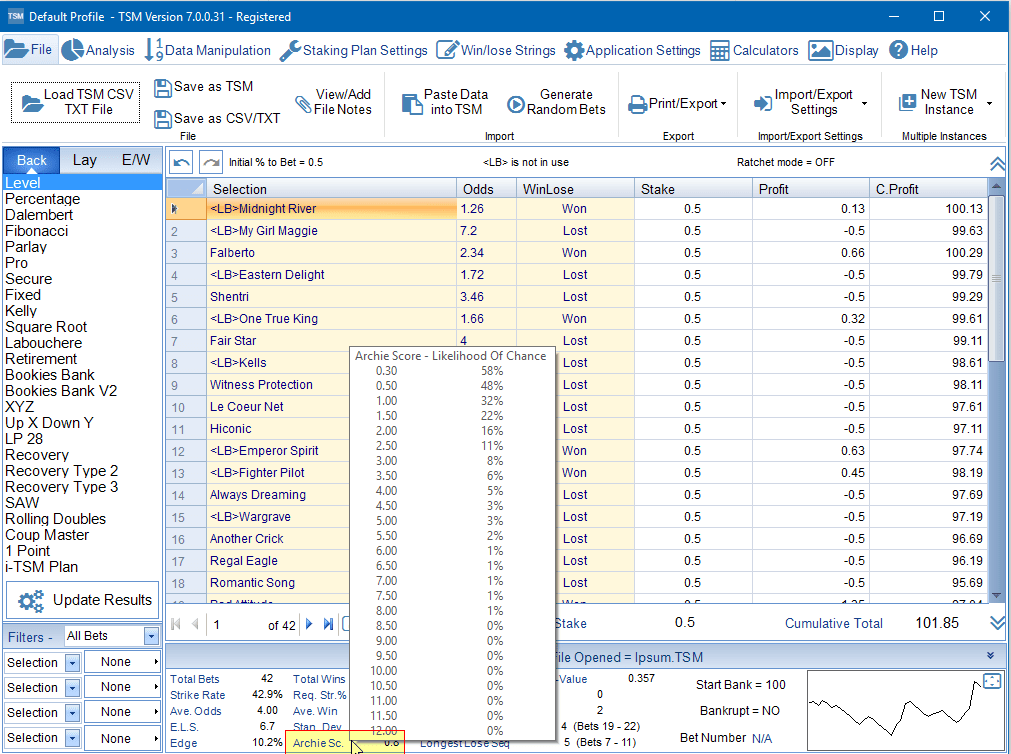# Archie Score – Chance or Fluke ?

How do you know if your betting results are down to chance or skill ?

This question has been explored in TSM from its inception in 2005. The very first TSM used the chi square formula to try and answer this question.

O=Observed frequency
E = Expected frequency
∑ = Summation
X2= Chi Square value

The Chi Square Formula was able to tell us on a very basic level whether the results were better than expected, expected or less than expected.

As time went on TSM changed to use the Monte Carlo Simulation which was a far better way of working out whether the results were better than expected, expected or less than expected when using staking plans. The Monte Carlo Simulation involves shuffling the data hundreds of times and recording the outcome for each staking plan. The Monte Carlo Simulation was able to provide important additional data such as the bankruptcy risk for each staking plan which was not available using the Chi Square Formula.

Now in 2016 we have decided to add what is called the Archie Score. This is based on the Chi Square Formula. By using a combination of both the Archie Score and Monte Carlo Simulations you should be able to make informed decisions when working out whether your betting results are a fluke or skill.

R = Runners (No. of Bets)
W = Winners (Winning Bets)
E = Expected number of Winning Bets
A = Archie Score

Working Example with only 5 bets so that the maths can be followed easily. In real life 5 bets is no where near enough to use any kind of statistics.

Expected Winners is simply the sum of the Probabilty Column. The probabilty is calculated by doing 1 divided by the decimal odds. So in this example the number of Expected Winners is 1.08.

We have 2 winners out of the 5 runners. Plug this in to the formula.

Archie Score = 5 x (2-1.08)² all divided by 1.08 x (5-1.08)

Archie Score = 4.168 / 4.233

Archie Score = 0.98

So what does this mean. People better at maths than me have produced a useful table. Looking at the table below it tells us that there is 32% probability that the results we obtained were down to chance.

In TSM the Archie Score is shown in the quick stats.Archie Score Location in TSM V7. Hover over the Archie Score Label to view the Archie Score Table.